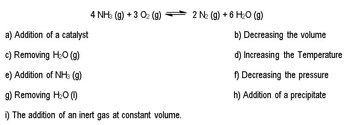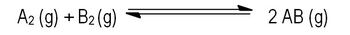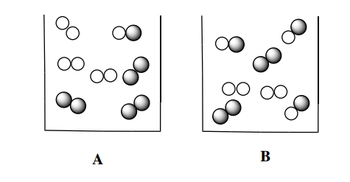## General Chemistry

Learn the toughest concepts covered in Chemistry with step-by-step video tutorials and practice problems by world-class tutors

16. Chemical Equilibrium

# Le Chatelier's Principle

Thermodynamics and Le Chatelier’s Principle
1
concept

## Thermodynamics1m
Play a video:
in this video, we're gonna continue our discussion of chemical equilibrium by taking a look at the equilibrium constant and its relationship to thermal dynamics. So here earlier, we learned that kinetics or chemical kinetics, study the rate at which are reacting changed into products. So basically, we're looking at the rate or speed of reactions in this chapter. We're now gonna learn about thermodynamics in thermal dynamics, deals with the direction that a chemical reaction at equilibrium will shift. So thermodynamics deals with the directional reaction takes whereas kinetics looks at the right or speed at which your reaction has, you need to take a look at both of them to get a complete idea of a chemical reaction. Now we're gonna stay here that late shuttlers principle solo shot Saliers Principle states that states that once a system which is a chemical reaction, that is that equilibrium is disturbed, it will do whatever it can to get back to equilibrium. So basically, you do something to try toe alternate alter the equilibrium state and the chemical reaction shifts to the left or to the right in order to minimize that effect. Now, knowing this, let's try to attempt to answer the questions below and look at the different things that we can do to a chemical reaction and as a result of that change, what happens to our chemical reaction?

According to Le Chatelier’s Principle if a system (chemical reaction) is at equilibrium and we disturb it then the system will readjust to maintain it’s equilibrium state.2
example

## The following is an endothermic reaction where Kc = 6.73 x 103.For each of the choices below predict in which direction the reaction will proceed9m
Play a video:
3
Problem

Consider the reaction below:

CH4 (g)  +  F2 (g)  ⇌  CF4 (g)  + HF (g)   H = + 38.1 KJ/mol

The following changes will shift the equilibrium to the left except one. Which one would not cause a shift to the left?

4
Problem

The following data was collected for the following reaction at equilibrium

2 A (g) + 3 B (g)  ⇌ C (g)

At 25 oC K is 5.2 x 10-4 and at 50 oC K is 1.7 x 10-7. Which of the following statements is true?

a) The reaction is exothermic.

b) The reaction is endothermic.

c) The enthalpy change, ΔH, is equal to zero.

d) Not enough information is given.

When looking at a chemical reaction we look at the two concepts of Kinetics and Thermodynamics to get a total view. However, remember that Le Chatelier’s Principle deals with Thermodynamics and not Kinetics.

5
Problem

Which direction will the following reaction (in a 10.0 L flask) proceed if a catalyst is added to the system?

CaCO3 (s)  ⇌ CaO (s) + CO2 (g)            Kp = 3.2 x 10-286
Problem

Consider the following gas reaction of A2 ( shaded spheres) and B2 ( unshaded spheres)

A2 (g)  +  B(g)  ⇌  2 AB (g)

Which container proceeds more to completion?

7
Problem
The water–gas shift reaction CO1g2 + H2O1g2Δ CO21g2 + H21g2 is used industrially to produce hydrogen. The reaction enthalpy is H = -41 kJ. (b) Could you increase the equilibrium yield of hydrogen by controlling the pressure of this reaction? If so would high or low pressure favor formation of H2(g)?